Ex 1.2

Chapter 1 Class 6 Knowing our Numbers
Serial order wise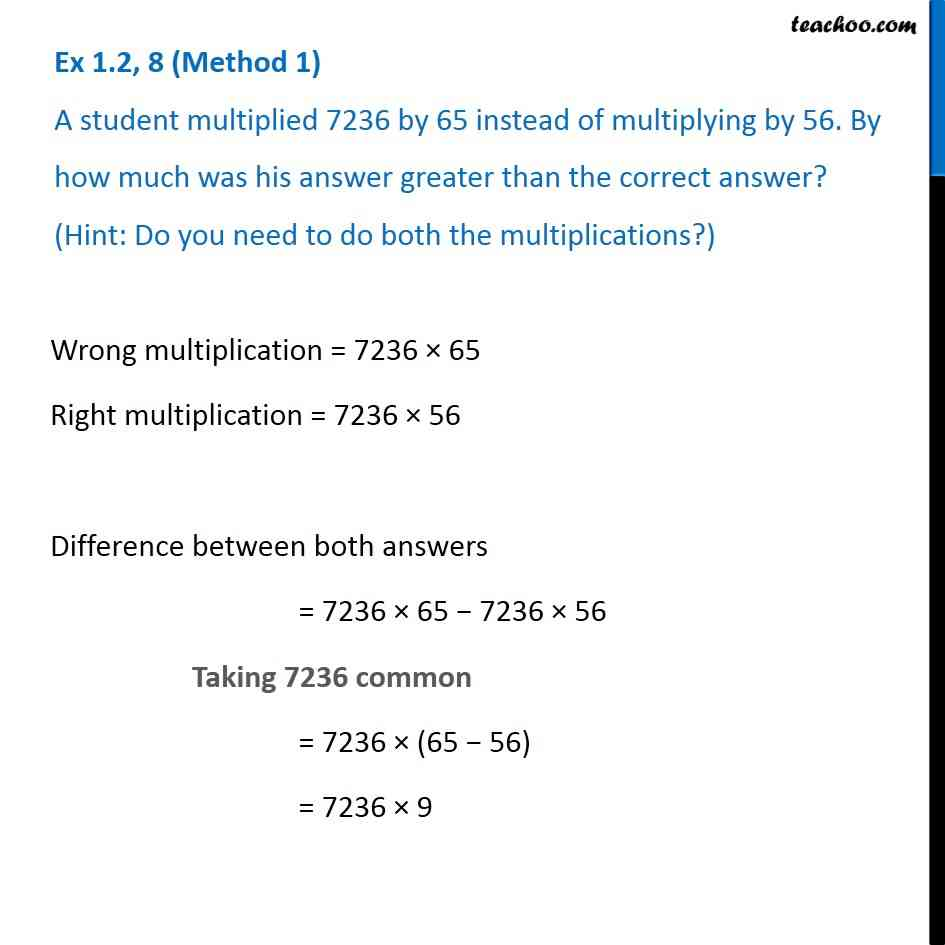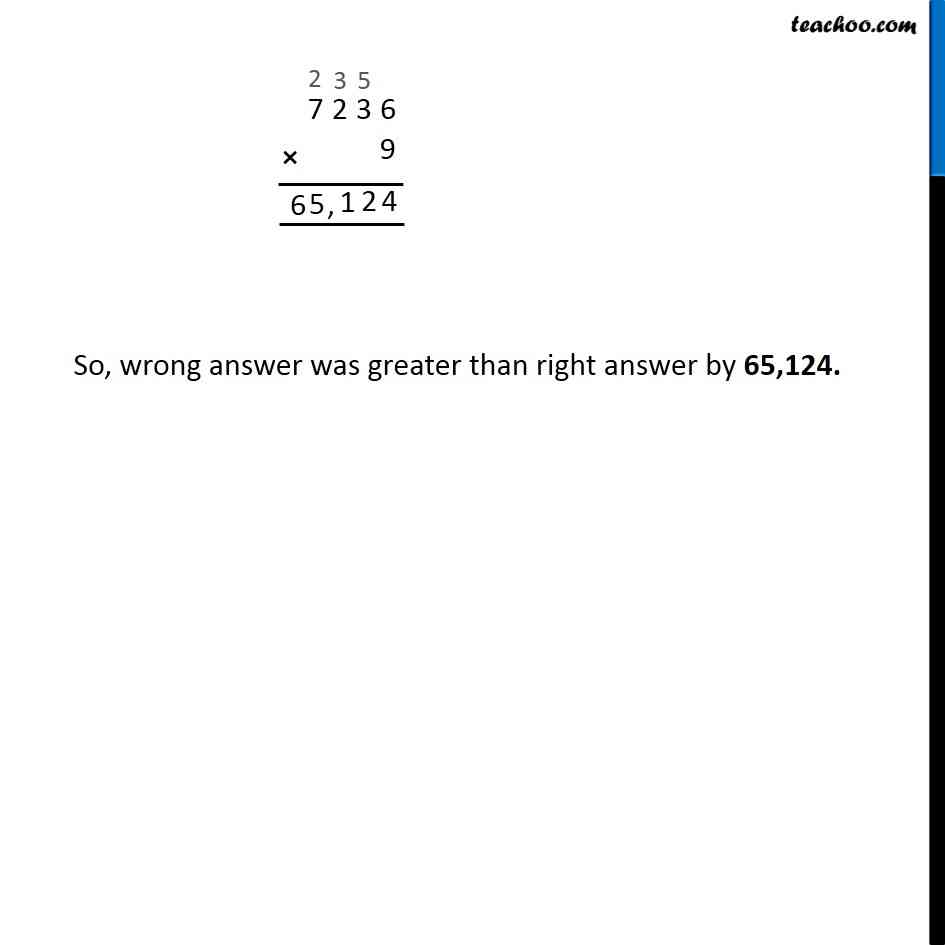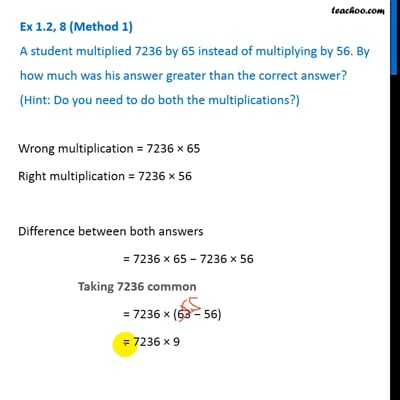This video is only available for Teachoo black users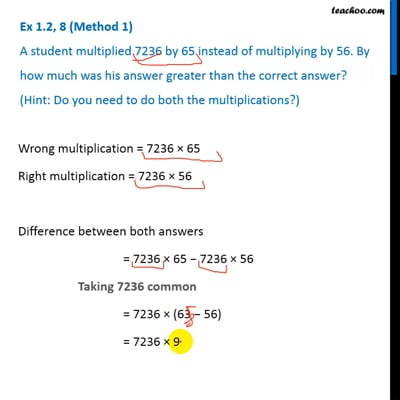This video is only available for Teachoo black users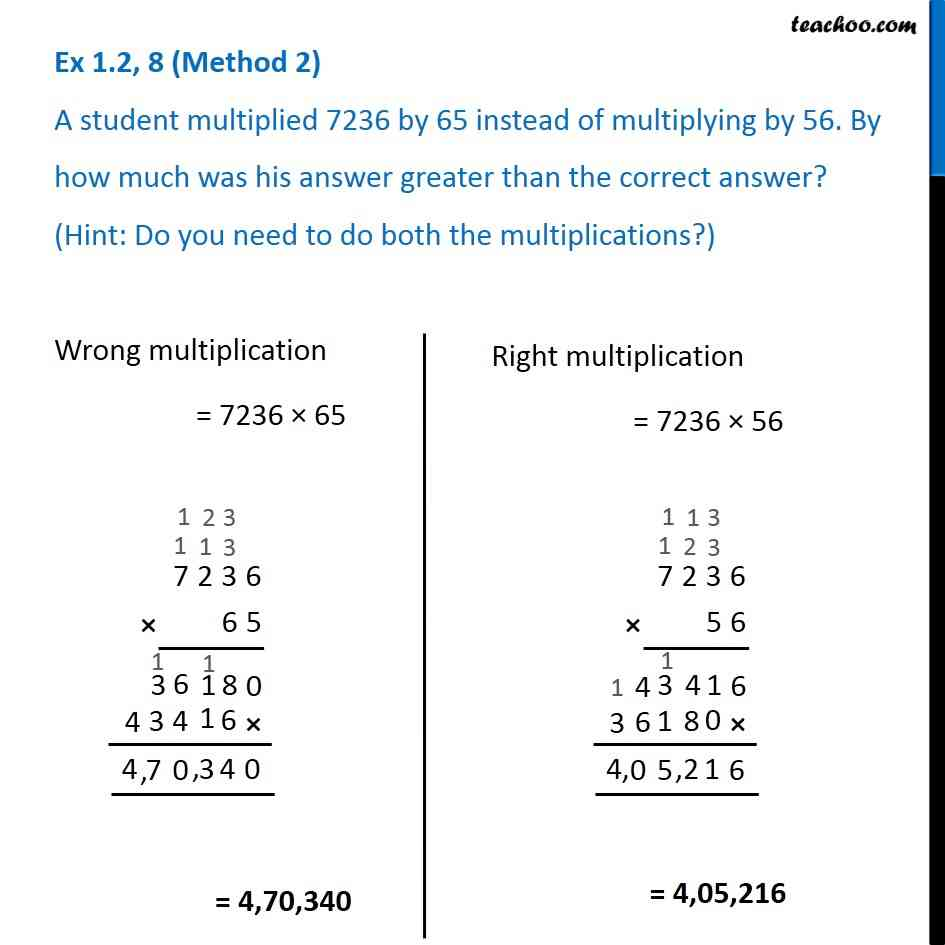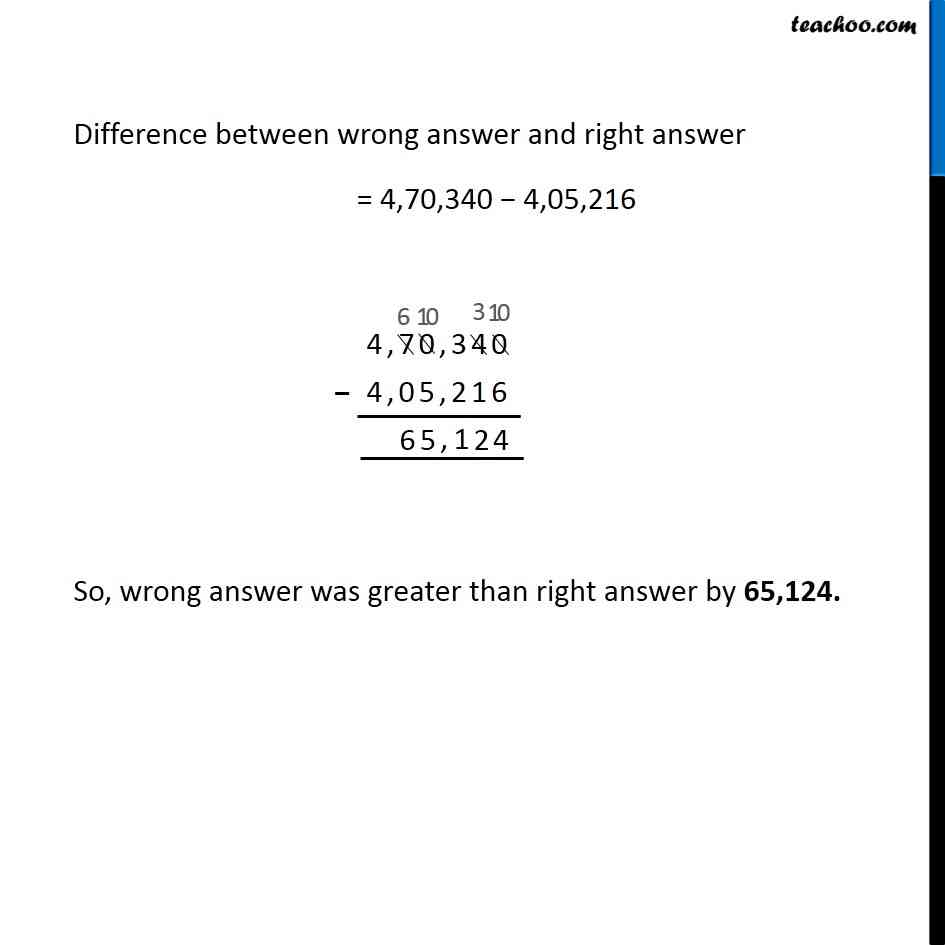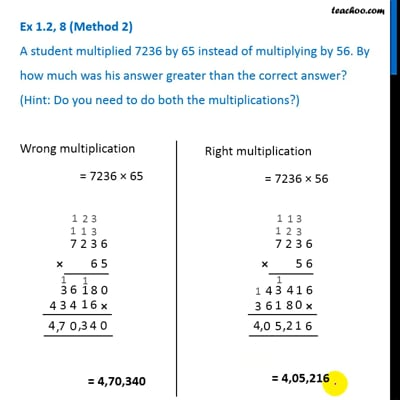This video is only available for Teachoo black users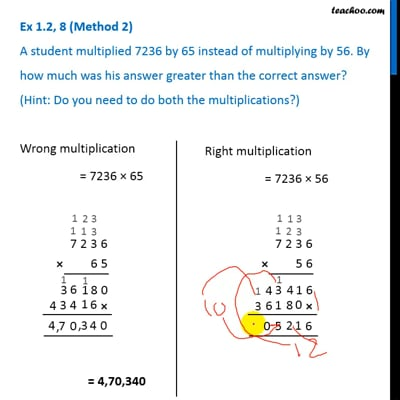This video is only available for Teachoo black users

Solve all your doubts with Teachoo Black (new monthly pack available now!)

### Transcript

Ex 1.2, 8 (Method 1) A student multiplied 7236 by 65 instead of multiplying by 56. By how much was his answer greater than the correct answer? (Hint: Do you need to do both the multiplications?) Wrong multiplication = 7236 × 65 Right multiplication = 7236 × 56 Difference between both answers = 7236 × 65 − 7236 × 56 Taking 7236 common = 7236 × (65 − 56) = 7236 × 9 So, wrong answer was greater than right answer by 65,124. Ex 1.2, 8 (Method 2) A student multiplied 7236 by 65 instead of multiplying by 56. By how much was his answer greater than the correct answer? (Hint: Do you need to do both the multiplications?)Wrong multiplication = 7236 × 65 Difference between wrong answer and right answer = 4,70,340 − 4,05,216 So, wrong answer was greater than right answer by 65,124.